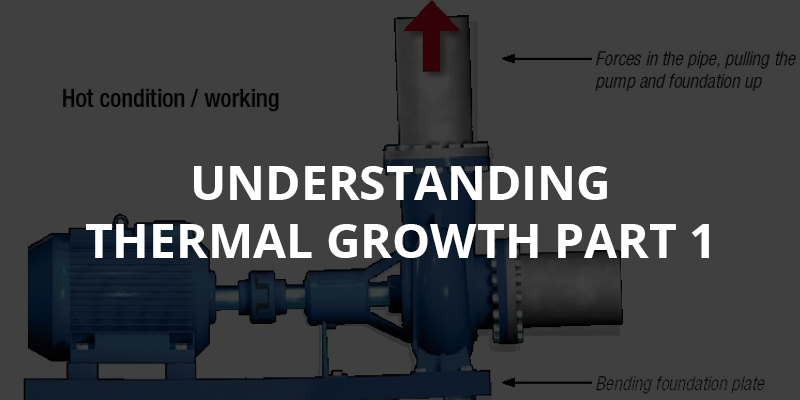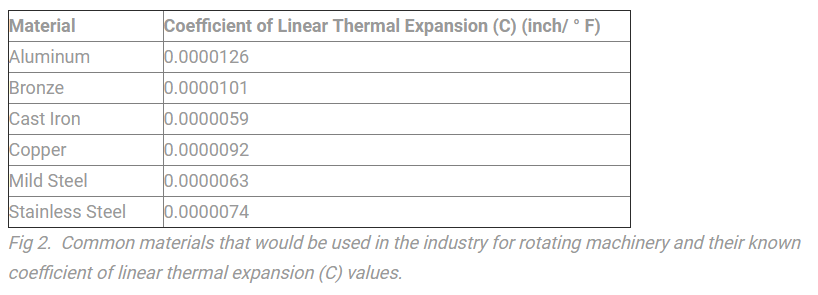## Understanding Thermal Growth in Your Rotating Machinery – Part 1

Thermal growth is an issue that is very much ignored because many think it too complicated to calculate and compensate for. Some are even unaware that it is an important issue that should be considered. However, many machinery manufacturers (for example, a Heat Pump Manufacturer) have to be aware of and take thermal growth into consideration when manufacturing such machines for home and commercial use. So our aim is to address this issue and hopefully simplify it so that it can be measured and compensated for on a more regular basis. We will do this over a three-part article – Part Two and Three will follow in the New Year. So excuse the pun but we want thermal growth to be the hot issue in 2016!Fig.1 An example of how hot liquids in the piping could pull the pump up, creating a twist or bent base frame as well as a misalignment between the shafts of the pump and motor.

As you know when something gets hot, it gets bigger; it expands. It happens to most things or materials but in this case we are only interested in metal, specifically iron, so that’s what we will work with. The coefficient of linear thermal expansion (C) is what is used to explain this expansion of material. For cast iron, it is 0.0000059″ per degree F. Looks like a small amount but it can add up – let me explain.The coefficient of the material (metal) to be measured is one of three things you need to know. The other two is how long the length of iron is and how hot it’s going to get. Let’s say it’s 12 inches long and will be heated to 100 F. Now, without writing out what looks like a complicated formula or equation in some textbooks, do this:

Take 0.0000059″ (C) and multiply it by 12″ (the length) and you get 0.00007″. Now multiply that by 100 (the temperature) and you should have 0.007″ thou (or 7.0 mils). Put simple, that length of cast iron will grow 7 thou (7.0 mils) if you heat it to 100 F.

That’s it – that’s thermal growth. Now apply it to your application. Let’s say you have a compressor that has a cast iron housing. The only area of this machine that we are interested in is in the plane of the machines feet. Imagine a line going up from the foot bolt through the machine but it stops at the center of the shaft. This is the length of the piece we want to measure. The reason is when this area grows and tries to move down it cannot because of the base, so it moves up and lifts the shaft. Anything above the shaft center line has room to grow so we do not care about this. The height from the base to the center of the shaft is 12 inches.

Now we take temperature measurements in the planes of the machines feet. The ambient (normal) temperature is 70 F and that’s what the motor is running at. So no thermal growth. The compressor is operating at 140 F so we have growth. What we need to know is the temperature change from normal (ambient) to running So, 140 F running less 70 F offline (140 – 70 = 70).  So our temperature change is 70 Fahrenheit. This is known as offline to running (OLTR) temperature.

This means that the compressor will rise (or grow) 0.0049″ – almost 5 thou (5.0 mils) – because 0.0000059″ (C) X 12″ (L) = 0.00007″. Now multiply 0.00007″ by 70 (T) and you get 0.0049″ (see Note 1 for a simple and common equation used in the industry).

To correct this is easy. You can put 5 thou (5.0 mils) under each of the four motor mounting feet so that as the compressor grows it will align the shaft centerlines. The other option is to set the compressor low by 5 thou (5.0 mils) – your choice.

This is just one simple example and the start of the thermal growth discussion that we can have. We would be happy to answer any questions you may have on the subject or even comment on information that you can send us so we can help you with this issue. Our goal is to make it simple at the start so we can all move forward together. Keep an eye out for Part 2 and 3 of Understanding Thermal Growth in Your Rotating Machinery where we will introduce other factors that can affect Thermal Growth.

Note 1: The formula or equation that is often used when calculating for thermal growth is T x L x C.

T = the temperature change of the material in Fahrenheit

L = the length in inches of the material

C = the coefficient of linear thermal expansion